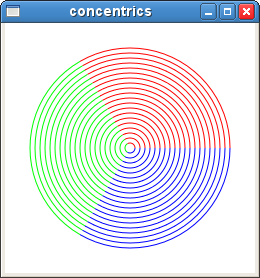Version 1 of Creating Circles and Arcs in the Gnocl Canvas

Updated 2010-03-05 01:43:49 by WJG

WJG (05/03/10) The gnocl canvas has no direct support for arc, is that odd? Not really, examination of the source for the Gnome Canvas shows that the ellipse is in fact a bezier circle. So, not only is this an approximation to a circle but the creation of arcs is going to be a nightmare. Ok, I've seen it done on Graphics apps such as animation systems using nurbs modelling, but we want to avoid curves as much as possible! Heres a simple, solution on the scripting side. Build a list of coordinates that generate a circle or arc and pass these to the gnocl::canvas object line command. This will create a suitable polyline. Here's a sample script that I've just puts together which does the job. Notice too, that the segments count for the arc is dependent upon the angle sweep. This could be modified to take into account the radius of the curve of the object if needed.

Here's the screenshot:So, here's the script:

#!/bin/sh
# the next line restarts using tclsh \
exec tclsh "\$0" "[email protected]"
package require Gnocl
package require GnoclCanvas

# x y co-ordinates of the centre of the arc
# r radius of the arc
# angle of start point
# angle of subtended by the arc # minium of step in the arc

proc rads {a}{return[expr \$a * 0.017453333] ;# ie PI/180}

proc poly_arc {x y r a1 a2 {s 4}} {
# calculate the number of segments
set j [expr \$a2 / 5]
if {\$j >= \$s } {set s \$j}
# calculate angle increment
set delta [expr \$a2 / \$s]
# create coordinates for the polyline
for {set da \$a1} {\$da <= [expr \$a1+ \$a2] } {incr da \$delta} {
set x2 [expr \$x + cos( [rads \$da] ) * \$r]
set y2 [expr \$y - sin( [rads \$da] ) * \$r]
lappend coords \$x2
lappend coords \$y2
}
return \$coords
}

set canv [gnocl::canvas -background white]

gnocl::window \
-width 250 \
-height 250 \
-child \$canv \
-title concentrics \
-onDelete exit

foreach {ang clr} {0 red 120 green 240 blue} {
for {set i 100} {\$i >= 5} {incr i -5} {
# draw the line
\$canv create line \
-coords [poly_arc 125 125 \$i \$ang 120] \
-fill \$clr \
-width 1 \
-tags "concentric"
}
}

gnocl::mainLoop

 Enter Category Here